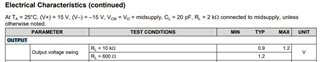If you have a related question, please click the "Ask a related question" button in the top right corner. The newly created question will be automatically linked to this question.

# OPA828: Output voltage swing

Part Number: OPA828

Hi team,

Could you kindly advise what the below parameter in the datasheet means ?Thank you and best regards,

Michiaki

• Tanii-san,

The OPA828 output voltage swing specification tells how close to the power supply voltage rails the output voltage can be for a specific load resistance RL. For example, if the OPA828 supplies are set to (V+) = 15 V, (V–) = –15 V, (TA = 25°C) and RL = 10 kΩ as in the first line, the OPA828 output will typically (TYP) be  0.9 V of either supply rail, or +15 V - 0.9 V = +14.1 V, and -15 V + 0.9 V = -14.1 V. Applying the MAX of 1.2 V would result in a worst case swing or +15 V - 1.2 V = +13.8 V and -15 V + 1.2 V = +13.8 V. The same simple calculations would be used for the RL = 600 Ω case, but there is only a TYP value of 1.2 V listed.

Do be aware that the output voltage swing test is what we refer to as a "slam" test; the output is being slammed up against the output rails. The output signal linearity will be degraded in that condition.

If the linear output range is needed, see the output swing range specified with the Open-loop voltage gan (Aol) specification in the table. For the RL = 10k Ω case the output voltage swing is then specified as (V–) + 1.5 V < VO < (V+) – 1.5 V. This is the output swing that should be relied upon to assure a highly linear output signal with RL = 10k Ω.

Regards, Thomas

Precision Amplifiers Applications Engineering